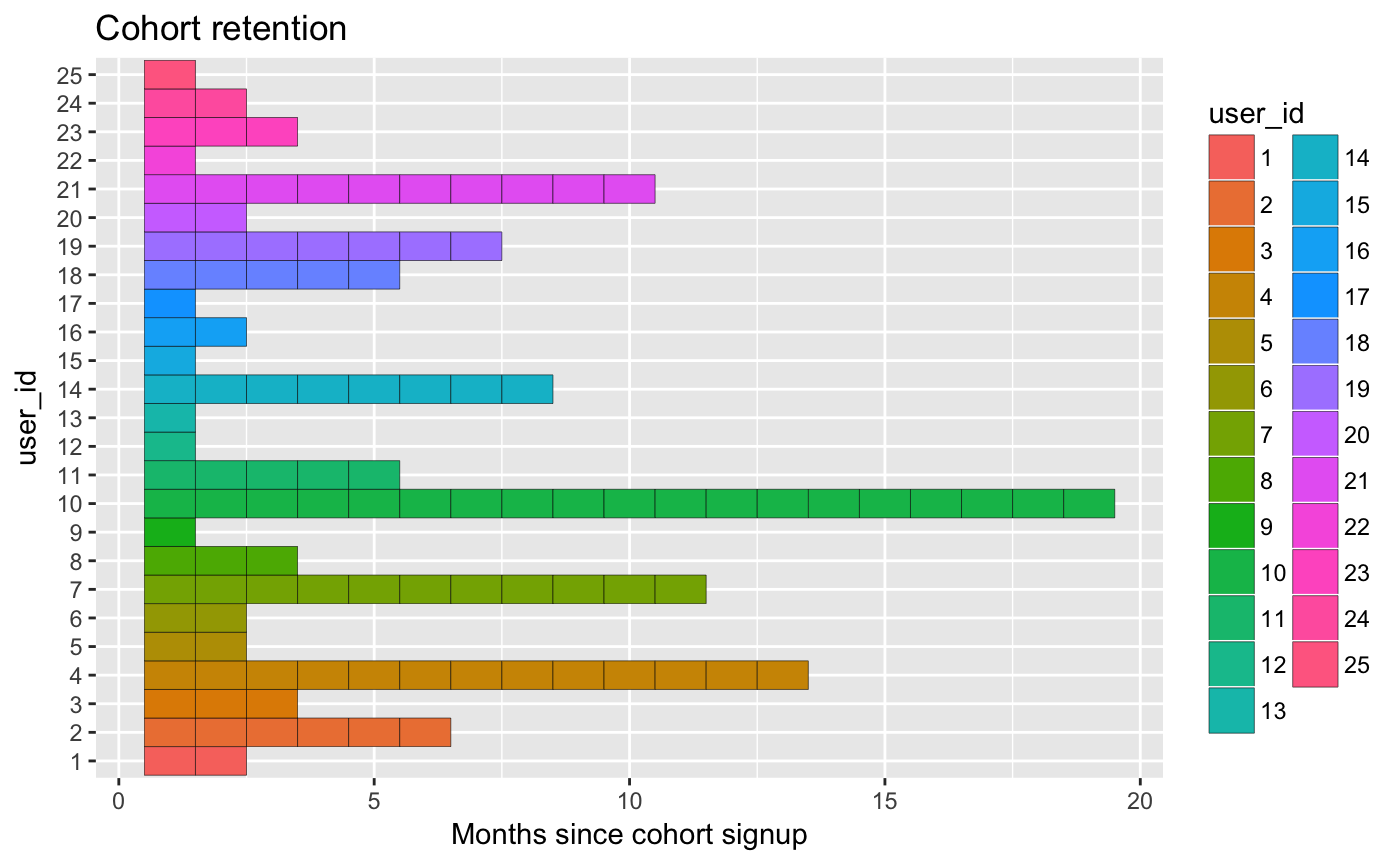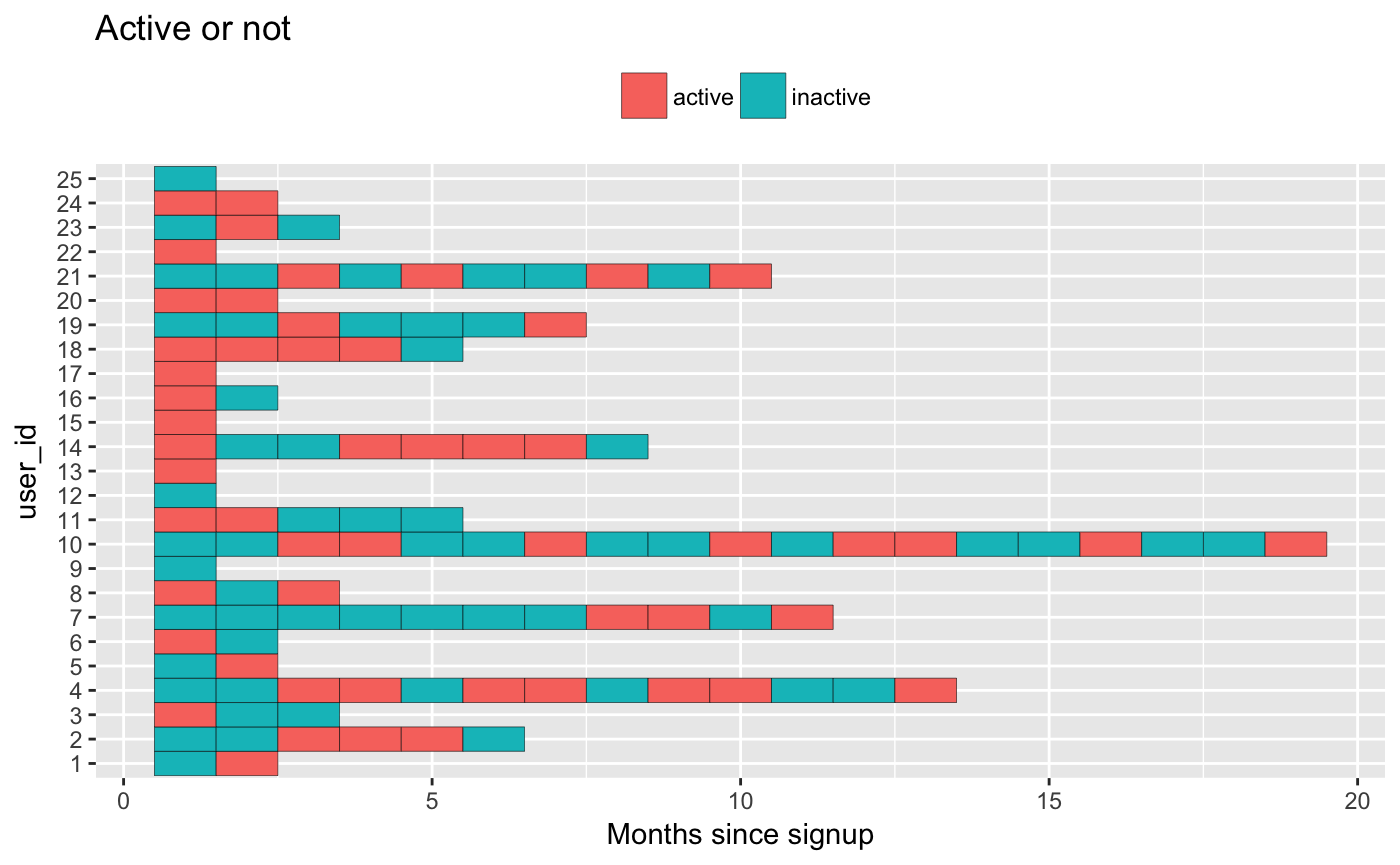The initial public offerings of Facebook and Twitter saw the public rise of the “active user” metric. Over the past few years Wall Street and Silicon Valley have had a near obsession with the metric.

“Active users” at its core is a count of user having initiated some website activity (often a subset of `GET`, `POST` http requests) usually reported by calendar month. Event logs are a common analytics data structure and the technique shown below is broadly suitable for learning the stories hidden in such a log of customer events.

At Pydata - Seattle 2015, Cameron Davidson-Pilon was advertising the lifetimes library for modeling phenomena like periodic purchases. He linked to a few academic articles that showed a new set of methods for customer-base analysis (e.g. event logs) offered by Drs. Peter Faber and Bruce Hardie of the Wharton School and London Business School, respectively. I noticed that one of the set of mixture models would be perfect for modeling active users.

In this notebook, I’ll share a few basics around modeling active users. It’s a work in progress. I expect future extensions to include building in of detail using Bayesian (“Bayesian survival analysis for “Game of Thrones”) and probabilistic programming techniques (pymc3, rstan). I’ll also leave model validation and projection to a future example. I think regression could be combined with this technique to yield interpretive insights.

This method starts with a simple story, that if believed, provides a coherent framework for modeling and ultimately projecting active users.

The story goes like this: when a user signs up for a service they’re given two coins. The first coin is flipped until they see “heads.” The number of times required to see “heads” is the number of months before the user abandons the site. The number of times can vary substantially between customers. The second coin is flipped for each month the user hasn’t abandoned the site. Each “heads” represents an active month.

Formally, this could be called a Beta-Geometric/Beta-Binomial (BG/BB) model. Drs. Faber and Hardie present a classic case of theoretically sound statistics substituting for a large quantity of engineering requirement by using a spreadsheet for calculations. Much like space and time, engineering and statistics are highly substitutive. When viewed this way and combined they can help solve otherwise challenging problems.

Now, I’ll build the first coin,

``````months_till_single_user_abandons <-  function(a, b) { rgeom(1, rbeta(1, a, b)) }
months_till_many_users_abandon <- function(users, a, b) {
replicate(users, months_till_single_user_abandons(a, b))
}``````

The geometric distribution has one parameter “p” where “p” is the probability of heads on a given flip. I mentioned customers vary significantly, so we want “p” to vary significantly. That’s where “a” and “b” come in, they’re from the beta distribution and have a mean of `a / (a + b)`.

Next I’ll assume a ten user cohort where each month there’s a roughly 1 in 6 chance each user abandons the site. The higher the total of “a” and “b” the more certain you are about the coin flip. It’s possible to accumulate cases and built up more and more certainty using counters.

I’ll simulate 25 users so we can visualize the steps as we go,

``````suppressPackageStartupMessages({
library(ggplot2)
library(dplyr)
library(tibble)
})
NUMBRER_OF_USERS <- 25
cohort <- tibble(months = months_till_many_users_abandon(NUMBRER_OF_USERS, 10, 50) + 1) %>%
mutate(user_id = seq_len(n())) %>%
group_by(user_id) %>%
do({ tibble(months = seq_len(.\$months), user_id = .\$user_id) }) %>% ungroup() %>%
arrange(user_id) %>% mutate(user_id = factor(user_id, levels = unique(user_id)))
cohort %>%
ggplot(aes(x = months, y = user_id, fill = user_id)) +
geom_tile(colour = "black") +
ylab("user_id") +
xlab("Months since cohort signup") +
ggtitle("Cohort retention")``````Now that we have the number of months until a user abandons the site, let’s model the number of months they’re active. This essentially involves flipping a coin for each month before abandonment.

I’ll build the second coin,

``````active_or_not <- function(a, b) {
ifelse(rbinom(1, 1, rbeta(1, a, b)), "active", "inactive")
}``````

Below is a graph marking “active” and “inactive” months. It’s important to note that the event(s) mapped to “active” vary widely company-to-company. However, allowing for extreme heterogeneity is what this type of model does best.

``````users_by_activity <- cohort %>%
rowwise() %>%
mutate(active = active_or_not(8, 10))
users_by_activity %>%
ggplot(aes(x = months, y = user_id, fill = factor(active))) +
geom_tile(colour = "black") +
ggtitle("Active or not") +
xlab("Months since signup") +
scale_fill_discrete(name = "") +
theme(legend.position = "top")``````Now we can group by months since the cohort signed up and count the active months,

``````users_by_activity %>%
filter(active == "active") %>%
count(months) %>%
ggplot(aes(x = months, y = n)) +
geom_bar(stat = "identity") +
ggtitle("Active users (retention aka 'stickiness')") +
xlab("Months since cohort signed up") +
ylab("Number of active users") +
scale_y_continuous(breaks = scales::pretty_breaks(10))``````
``Grouping rowwise data frame strips rowwise nature``